# 读论文——On2Vec：基于嵌入的本体群体关系预测

## 研究背景

### 问题

$$S_{r}(\mathbf{s}, \mathbf{t})=|\mathbf{s}+\mathbf{r}-\mathbf{t}|$$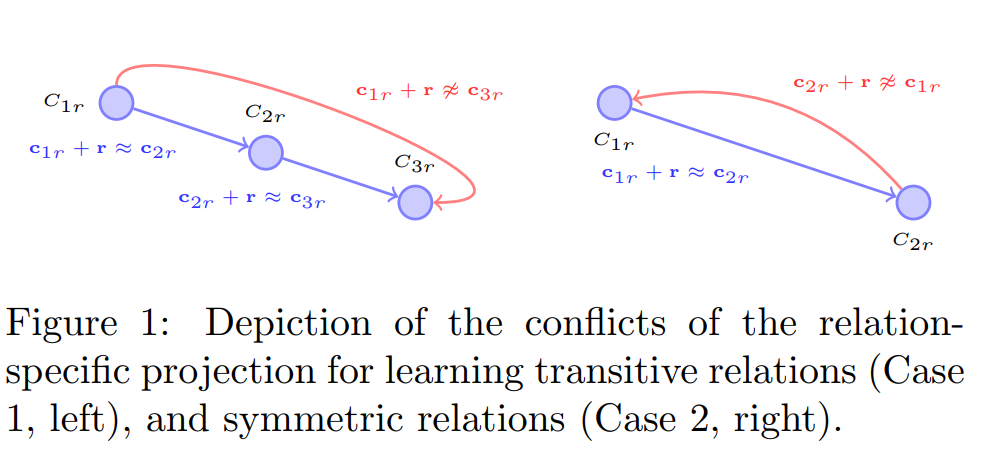• Case1：$\boldsymbol {A,B,C}$是三个概念$A,B,C$的嵌入，假设
$$\boldsymbol {A+r\approx B，B+r\approx C}$$
即$r$是一种具有传递性的关系，按照传递性的原则应当有：
$$\boldsymbol {A+r\approx C}$$
然而事实上：
$$\boldsymbol {A + r\ne C}$$
这个结论很容易就能从图中观察出来。

• Case2：$\boldsymbol {E,F}$是两个概念$E,F$的嵌入，假设：
$$\boldsymbol {E+r\approx F}$$
且$r$为对称性关系，则应有：

$$\boldsymbol {F+r\approx E}$$

然而事实上：
$$\boldsymbol {F+r\ne E}$$
这是因为向量$\boldsymbol r \ne 0$。

### 改进

1. Component-specific Model （组件特定模型）将概念和关系编码嵌入低维空间，且不丢失相关属性；
2. Hierarchy Model （层次模型）集中处理层级关系。

## 技术路线

### 符号及其含义

$G(C,R）$ 一个图谱
$C$ 一系列的概念
$R$ 一系列语义关系
$T=(s,r,t)$ 一个三元组
$\boldsymbol s$ vectors of source
$\boldsymbol r$ vectors of relation
$\boldsymbol t$ vectors of target
$R_{tr}$ 传递关系 如isConnectedTo
$R_s$ 对称关系 如isMarriedTo
$R_h$ 层级关系
$R_r$ 将粗概念划分为细概念的细化关系 如hasChild
$R_c$ 将细概念划分到粗概念的强制关系 如isLocatedIn
$R_o$ 其他关系

$$given: r \in R_{tr} \ c_1,c_2,c_3 \in G \ if:(c_1,r,c_2),(c_2,r,c_3) \in G \ then : (c_1,r,c_3) \in G$$

### 建模

#### Component-specific Model

##### 原理

$$S_{d}(T)=| f_{1, r}(\mathbf{s})+\mathbf{r}-f_{2, r}(\mathbf{t}) \mid$$

The forms of $f_{1,r}$and $f_{2,r}$ are decided particularly by the techniques to differentiate the concept encoding under different contexts of relations.

##### 优化

CSM的目标是最小化能量函数，也就是增加所有三元组的可信度。和别的模型选择实体做负面样本不一样的是，CSM选择关系来做负面的样本，由此提出loss：

\begin{aligned} S_{\mathrm{CSM}}(G)=& \sum_{(s, r, t) \in G}\left[\left|f_{1, r}(\mathbf{s})+\mathbf{r}-f_{2, r}(\mathbf{t})\right|\right.\left.-\left|f_{1, r}(\mathbf{s})+\mathbf{r}^{\prime}-f_{2, r}(\mathbf{t})\right|+\gamma_{1}\right]_{+} \end{aligned}

#### Hierarchy Model

##### 原理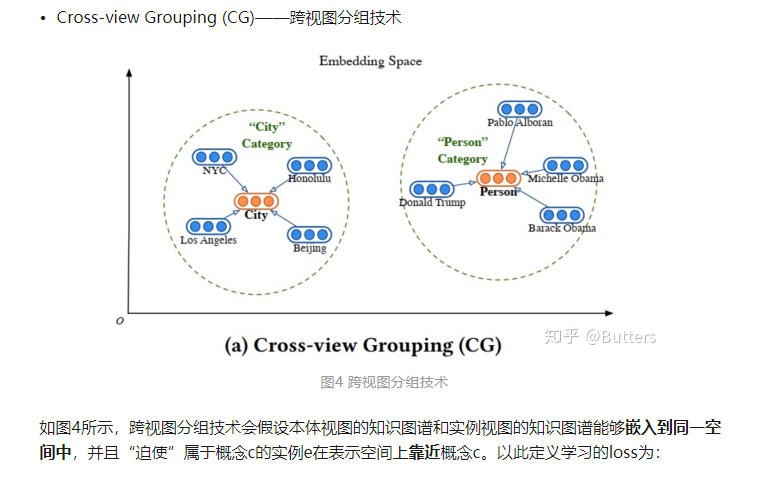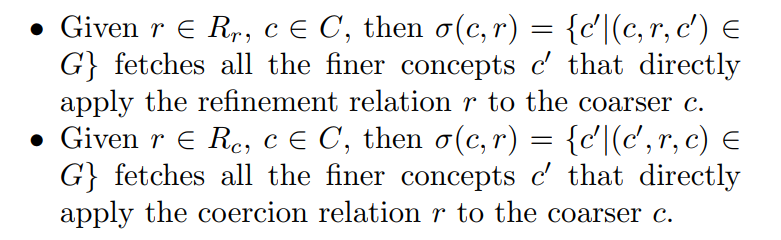• 设$(c_1,isA,c_2),(c_2,isA,c_3),(c_4,isA,c_3),(c_5,isA,c_3) \in G$

• 则有$\sigma(c_3,isA) = [c_2,c_4,c_5]$

• 虽然$(c_1,isA,c_2),(c_2,isA,c_3)$得到$(c_1,isA,c_3)$，但是这里认为$c_1$并不与$c_3$直接相关，故$c_1 \notin\sigma(c_3,isA)$

\begin{aligned} S_{h m}(G) &=\sum_{r \in R_{r}} \sum_{s \in C} \sum_{t \in \sigma(s, r)} \omega\left(f_{1, r}(\mathbf{s})+\mathbf{r}, f_{2, r}(\mathbf{t})\right) \ &+\sum_{r \in R_{c}} \sum_{t \in C} \sum_{s \in \sigma(t, r)} \omega\left(f_{2, r}(\mathbf{t})-\mathbf{r}, f_{1, r}(\mathbf{s})\right) \end{aligned}
$f_{1,r}和f_{2,r}$就是上一节写到的调整函数。$\omega (x)$是用来计算两个向量的相似度的单调递增的函数，算法中直接计算两个向量的余弦距离，余弦距离越小，余弦相似度越高。第一行计算的是所有直接细化关系的三元组的偏差，第二行计算的是所有直接强制关系的三元组的偏差，相加得到整个图谱的偏差。

##### 优化

\begin{aligned} S_{\mathrm{HM}}(G) &=\sum_{r \in R_{r}} \sum_{s \in C} \sum_{t \in \sigma(s, r) \wedge \ t^{\prime} \notin \sigma(s, r)} S_{h r} \ &+\sum_{r \in R_{c}} \sum_{t \in C} \sum_{s \in \sigma(t, r) \wedge \ s^{\prime} \notin \sigma(t, r)} S_{h c} \end{aligned}

$s’$和$t’$在这里做负面样本，其中

$$S_{h r}=\left[\omega\left(f_{1, r}(\mathbf{s})+\mathbf{r}, f_{2, r}(\mathbf{t})\right)-\omega\left(f_{1, r}(\mathbf{s})+\mathbf{r}, f_{2, r}\left(\mathbf{t}^{\prime}\right)\right)+\gamma_{2}\right]_{+}$$

$$S_{h c}=\left[\omega\left(f_{2, r}(\mathbf{t})-\mathbf{r}, f_{1, r}(\mathbf{s})\right)-\omega\left(f_{2, r}(\mathbf{t})-\mathbf{r}, f_{1, r}\left(\mathbf{s}^{\prime}\right)\right)+\gamma_{2}\right]_{+}$$

### 学习流程

$$J(\theta)=S_{\mathrm{CSM}}+\alpha_{1} S_{\mathrm{HM}}+\alpha_{2} S_{\mathrm{N}}$$

• $\theta$ 是包括嵌入向量和投影矩阵的一系列的参数的集合
• $\alpha_{1} S_{\mathrm{HM}}$中的$\alpha_1$用来调整两个模型的权重
• $\alpha_1S_N$中的$\alpha_1$是一个大于零小于等一的数，$S_N$用来对嵌入和投影施加约束，防止出现向量趋向于无限大的情况。其形式如下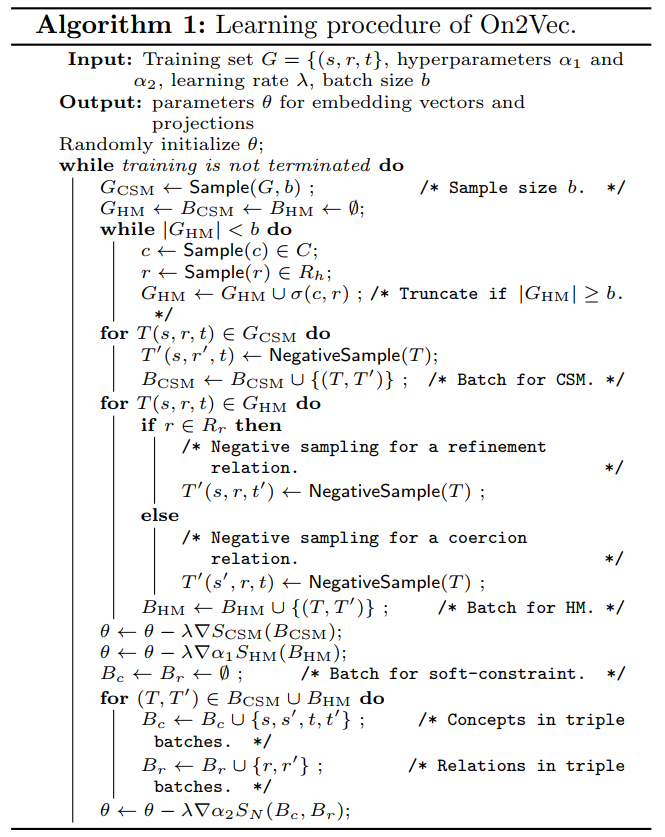### 数据集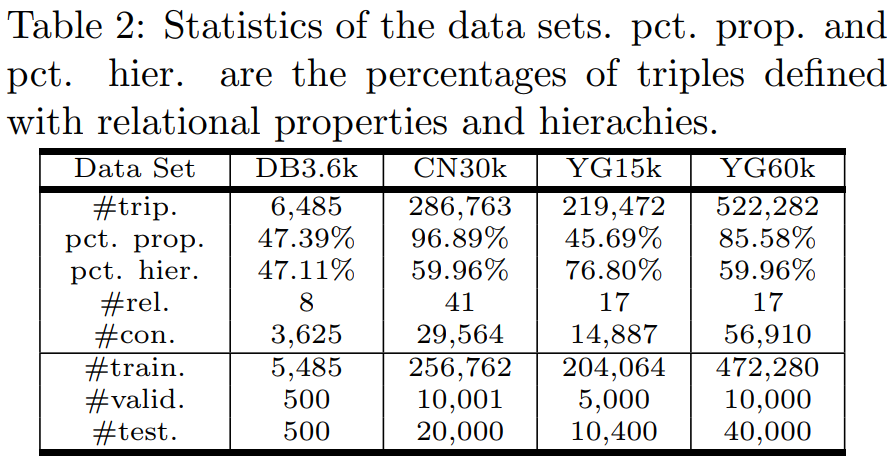目录